# Multicolor Lighting Employs High-Power Drivers for RGB LEDs

### Abstract

This article looks at the design of high-power LED drivers suitable for powering multicolor lighting systems that include high-brightness LEDs (HB LEDs). The circuit presented as an example drives the RGB LEDs found in decorative, architectural, entertainment, and stage lighting.

A similar article appeared in Korea in the March, 2009, issue of Display magazine.

Next-generation architectural and decorative lighting achieves a wide range of color by mixing appropriate proportions of output from red, green, and blue LED chips. The series-connected chips in such high-brightness, multichip LEDs feature typical forward voltage drops of 22V to 36V, while drawing 1A to 2A currents. The LED driver featured in Figure 1 delivers 2A to a multichip LED module whose forward voltage can range up to 36V. This circuit drives only one color of the RGB LEDs, so three such drivers are needed to drive all three colors.

Because the light output from an LED is not a linear function of its forward current, brightness levels are adjusted via PWM control rather than by controlling the LED's current amplitude. That is, each LED is driven with a constant current that is pulse-width modulated to control the light output. The IC controller shown uses average-current-mode control to implement this LED drive with a minimum of external components.More detailed image
(PDF, 264kB)
Figure 1. This circuit drives one high-brightness LED of an RGB chipset. Three such circuits drive a typical multicolor lighting system.

### Detailed Description

To efficiently deliver the current, this LED driver employs boost topology in continuous-conduction mode (CCM), using average-current-mode control to step up the input voltage and drive a constant current through the LED load. A single device (MAX16821B) operating at 300kHz controls the boost converter operation. Because boost converter topologies provide a direct path between the converter's input and output, you must ensure that the LED string's minimum forward voltage exceeds the maximum input supply voltage.

The LED load connects across the boost converter output through a MOSFET (Q1) and a current-sense resistor (R13). Q1 turns on the LED current during PWM ON, and turns it off during PWM OFF. While differentially sensing the voltage across R13 (which represents current through the LED), the IC rejects any common mode noise and provides a ground-referenced output with gain of 6V/V at the DIFF pin. This amplified current-sense signal is then compared against a 0.6V reference by an internal voltage-error amplifier. In effect, the 6V/V gain of the differential current-sense amplifier reduces the current-sense reference from 0.6V to 0.1V. This reduction improves efficiency, because R13 drops only 0.1V at the rated load current.

The average-current-mode control employed by the boost converter in this application uses two feedback loops to control the LED current. An outer voltage loop senses the LED current, compares it with a reference, and generates an amplified error signal available at EAOUT (pin 17). An inner current loop looks at the error amplifier's voltage output, and controls current through the inductor (L1) accordingly. The error amplifier output also determines the average inductor current necessary to drive the LED current programmed by R13, i.e., the LED current that produces a drop of 0.1V across R13.

A second current-sense resistor (R15) for inductor current resides in the inductor's return path. A differential current-sense amplifier with gain of 34.5V/V, internal to U2, amplifies the current-sense voltage across R15 while rejecting any common-mode noise. The current error-amplifier then compares this output with the output of the voltage-error amplifier, and thereby generates an error signal for the internal average-current control loop. That amplified error signal is compared with an internal oscillator ramp to generate the PWM signal (at DL, pin 3) for driving MOSFET Q2. High gain in the current-error amplifier enables the circuit to produce the average inductor-current values demanded by the voltage loop (within the maximum limit allowed), with very low error.

For a given input supply voltage and LED forward voltage (ignoring voltage drops in the switch, diode, sense resistor, etc.) the boost converter's CCM operation determines (fixes) the duty cycle for the PWM switch. A fix of duty cycle corresponding to a required LED current then determines the required average current through the inductor. The voltage loop causes the inner-current loop to drive this average inductor current as necessary to deliver the required LED current. Both control loops should be compensated separately for stable operation. The design of this compensation is explained in detail in the Feedback compensation section.

### Converter Design

You need the following parameters to design the converter:

• Input supply-voltage range: 9V to 15V
• Maximum LED forward voltage: 33V
• LED current: 2A
• Switching Frequency: 300kHz. (Lower frequencies increase the filter cost; higher frequencies reduce efficiency and increase the EMI. Considering these factors, the optimal switching frequency is about 300kHz.)
Calculate the maximum ON duty cycle for Q2 using the following equation: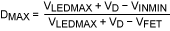, where VLEDMAX is the maximum LED forward voltage (which should also include the voltage drops across MOSFET Q1 and sense resistor R13), VD is the drop across rectifier diode D1, VINMIN is the minimum input supply voltage, and VFET is the average voltage across MOSFET Q2 when ON. For this application, DMAX = 0.74.

To select the inductor (L1), you must consider its value and the required peak current rating. Use the following equation to calculate the maximum average inductor current (ILAVG):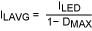.

To determine the peak inductor current (ILPEAK), note that a certain amount of ripple current flows through the inductor, depending on the inductor's value and the switching frequency. You should therefore assume a maximum of ±20% peak-to-peak ripple (ILP-P) in the inductor current. Since ILP-P is ±20% of the average inductor current ILAVG,.

Using known values in the above equations yields ILAVG = 7.7A, and ILPEAK = 9.24A.

Next, calculate the minimum inductance value LMIN, with the inductor current ripple set to the maximum value:, where FSW is the switching frequency.

Using known values in the above equation yields ILMIN = 7.05µH. After adding a ±20% tolerance on the inductance value, we select a standard value of 10µH.

Resistor R15 senses the average current through the inductor. A current that drops 25.7mV (minimum) across R15 is the maximum current permitted through the inductor by the average-current control loop. This feature, which protects external devices in the event of an overload, is implemented by clamping the maximum value of reference voltage applied to the current-error amplifier.

The value of R15 should ensure that the voltage across it is less than 25.7mV at the maximum possible inductor current. For this application, the maximum voltage across R15 during normal operation is selected to be 24mV. You can calculate the value for R15 using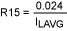, where the use of known values yields R15 = 3.11mΩ. Accordingly, we select a 3mΩ resistor for this application.

### Filter Capacitors

To calculate a value for the output capacitor, COUT (the parallel combination of C6, C7, C8 and C9), use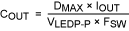, where VLEDP-P is the peak-to-peak ripple in the boost output voltage. This peak-to-peak ripple combined with the LED dynamic impedance at rated current decides the LED ripple current. To preserve chromaticity and long life for the LED, the LED ripple current should be kept below 10% of the average current. Substituting known values in the above equation yields COUT = 17µF, which is approximated in the circuit using 4.7µF, 50V ceramic capacitors.

Calculate a value for the input capacitor CIN (the parallel combination of C3, C4, and C5) using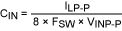, where VINP-P is the peak-to-peak input ripple voltage, which for this application is taken as ±0.4% of the input voltage. Substituting known values in this equation yields CIN = 22.3µF, which is approximated at the input (left end of L1) with three 10µF, 25V ceramic capacitors as shown.

### Average-Current Control Loop

To ensure stability in the average-current control loop, you should set the gain of the current-error amplifier below a certain value (for frequencies near the switching frequency). The reason is as follows: because the inductor current measured across R15 is declining during the Q2 OFF time, it has a negative slope during this period. This negative slope is amplified and applied to the input of the error amplifier, further amplified by the current error amplifier, and applied as a positive slope at the PWM comparator input.

For the current loop to be stable, this positive slope must not exceed the positive slope of the ramp signal applied at the other input of the PWM comparator. That condition puts an upper limit on the total gain of the inductor-current signal (at the switching frequency) before it reaches the PWM comparator. At lower frequencies the total gain can be higher, to allow the average inductor current to settle accurately to its programmed value in the steady state.

The IC shown (U2) allows you to satisfy the condition for stability by controlling the gain of the current error amplifier. The following equation lets you calculate the maximum gain allowed (at the switching frequency) to ensure loop stability for this amplifier: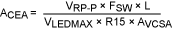, where VRP-P is the peak-to-peak value of the internal ramp (2V), L is the inductance value of L1, and AVCSA is the differential gain of the current-sense amplifier (34.5V/V). Substituting known values in this equation yields ACEA = 1.75V/V.

The internal current error amplifier is a transconductance type with gain of 550µs (550µA/V). Resistor R10, connected to the error amplifier output at CLP (pin 16), controls the gain of the current error amplifier at the switching frequency. The value of R10 is, where a substitution of known values yields R10 = 3.18kΩ. For this application, an acceptable standard value is 3.16kΩ.

If R10 connects directly to GND, then the gain of the current error amplifier is 1.75V/V for all frequencies below the 3dB frequency of the current error amplifier. For the loop to be stable, on the other hand, the overall gain needs to be at 1.75V/V only near the switching frequency. Even if gain is high at lower frequencies, however, the down slope in inductor current is not amplified, because the inductor current ripple does not have frequency components below the switching frequency.

A zero placed in the transfer function of the current error amplifier causes the gain of the current loop to be flat above the zero frequency (at 1.75V/V), and increase inversely with frequency below the zero. The zero frequency is determined by C11 and R10. For this application, an optimal position for the zero is at one twelfth of the switching frequency, which allows the average inductor current to settle quickly to the programmed value.

To place the zero at one twelfth of the switching frequency, you can calculate the value of C11 as follows: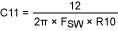. Substituting known values in this equation yields C11 = 1.99nF, for which a reasonable approximation in this application is 2.2nF.

C10 introduces a high frequency pole at the switching frequency, which serves to attenuate any undesirable noise spikes caused by switching:. Substituting known values in this equation yields C10 = 152pF, for which 180pF is a reasonable approximation.

### Voltage Control Loop

LED current is controlled by using feedback to maintain a constant voltage across the LED current-sense resistor (R13). Producing a constant value that depends on the value of the LED current and switching duty cycle, the voltage control loop generates an input reference for the current control loop, which in turn programs the average inductor current. Comparing the drop across R13 (the LED current-sense resistor) with an accurate 100mV reference, the voltage-error-amplifier amplifies the difference and generates a reference voltage corresponding to the required value of average inductor current. The following equation calculates a value for R13 based on the LED current output: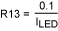, where ILED is the LED current (2A in this application), and 0.1V is the feedback reference for the voltage control loop. Substituting known values in this equation yields R13 = 0.05Ω. The power rating for this resistor should be more than ILED² times the value of R13.

Because the boost converter operates in continuous conduction mode, there exists a right half plane (RHP) zero in the power-circuit transfer function. This zero adds a 20dB/decade gain and a 90° phase lag, which is difficult to compensate. The easiest way to avoid this zero is to roll off the loop gain to 0dB (with -20dB/decade slope), at a frequency less than that of the RHP zero. For a boost converter, the worst-case RHP zero frequency (FZRHP) is given by the following equation:. Substituting known values in this equation yields FZRHP = 17.7kHz.

The control loop for average current converts the double-pole, 2nd-order system formed by the inductor and output capacitor COUT to a 1st-order system having a single pole consisting of the output filter capacitor and the dynamic impedance of the output load (ΔV/ΔI for the LED at rated current). The frequency of the output pole formed by the output filter capacitor and the output load dynamic impedance is given by, where RLD is the dynamic impedance of the LED load (4.5Ω for the LEDs used in this application). Substituting known values in this equation yields FP2 = 1.88kHz.

The DC gain of the voltage control loop—from the output of the voltage error amplifier to the output of the differential voltage amplifier, at maximum duty cycle—is given by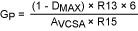, where 6V/V is the gain of the differential voltage amplifier internal to U2 in Figure 1. Substituting known values in this equation yields GP = 0.75V/V.

To compensate the voltage control loop (so the loop is stable and has sufficient phase margin), note that the loop crossover frequency (FC) should be below one fifth of the RHP zero frequency. To get a good phase margin in this application, we select the crossover frequency to be one tenth of the RHP zero frequency:. Substituting known values in this equation yields FC = 1.77kHz.

The transfer function for the voltage error amplifier has a dominant pole (FP1), a zero (FZ1) to compensate for the output pole FP2, and a high-frequency pole (FP3). The compensation zero (FZ1) is placed at the output pole frequency. Use the following equation to calculate the value of gain (at FZ1) in the voltage error amplifier that causes the total loop gain to cross 0dB at FC:. Substituting known values in this equation yields AEA1 = 1.25V/V.

Resistors R14 and R12 determine the gain AEA1:. Setting R12 to the arbitrary value of 2.2kΩ in this equation yields R14 = 2.75kΩ.

C14 and R14 determine the location of the compensating zero FZ1. Calculate the value of C14 as follows:. Substituting known values in this equation yields C14 = 30.8nF, for which a 100nF value is reasonable. The higher value improves PWM performance, and maintains the charge in C14 by opening switch Q3 during PWM OFF. This action is explained in the section Low frequency PWM dimming.

C12 places a high frequency pole (FP3) at half the switching frequency. Calculate this C12 value as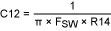. Substituting known values in this equation yields C12 = 386pF, for which a 470pF value is reasonable.

### PWM Dimming and LED Protection

The LED is dimmed by a low-frequency PWM signal connected to the PWMDIM input (an external signal applied to the Figure 1 circuit). The amplitude of this PWM signal can be from 3V to 10V, and the frequency can be up to 2kHz. An external MOSFET (Q1) in series with the LED in this circuit enables the LED current to turn on and off rapidly and settle quickly. Q1 turns on during PWM ON, and turns off during PWM OFF. When the LED is turned off, U3 pulls down CLP (U2 pin 16) to disable the PWM switching and turn off Q2.

The small-signal MOSFET Q3 performs an important function that affects the response time of the LED current-control loop during PWM dimming. It turns off during the PWM OFF time, isolating C12/C14 so their charge remains unchanged during the OFF time. When the PWM turns back ON, the output of the voltage error amplifier can therefore assume the previous steady state value almost instantly, thereby ensuring that the LED current reaches its set value almost as soon as the LED turns on.

A general-purpose op amp (U1) protects the LED by blocking its current when the LED temperature reaches 85°C. Temperature is sensed by an EPCOS® NTC resistor. Mounted on the LED board and assuming a value of 10kΩ at 25°C, it enables the op amp to control U2's EN input (pin 11), causing the LED to turn off at 85°C and back on at 75°C.

Without overvoltage protection, the boosted output voltage can increase to unsafe levels in response to an open-circuited LED. The circuit of Figure 1 guards against against this fault by turning off the converter when the boosted output voltage reaches 33.5V. It implements this protection by turning off the PWM switching when the voltage at U2's OVI input (pin 15) crosses 1.276V—a threshold set by the R5/R7 resistive divider to correspond to the desired overvoltage limit of 33.5V. To maintain accuracy for the overvoltage threshold, select an R7 value below 25kΩ. Then, use the following equation to calculate an R5 value corresponding to the desired overvoltage threshold:, where VOVT is the desired threshold.

The waveforms of Figure 2 (9V supply) and Figure 3 (15V supply) show that by adjusting the inductor current, you can maintain a constant LED current at different supply voltages. The pc layout for a typical implementation is shown in Figure 4. To obtain a parts list and photos of the 4-layer circuit board, download (PDF).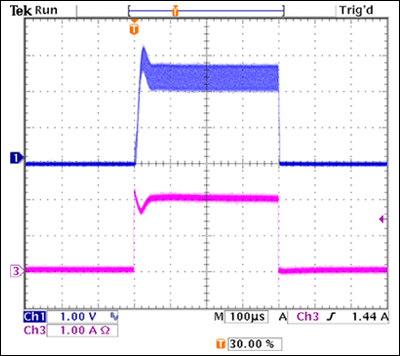Figure 2. The Figure 1 circuit operating on a 9V input supply produces these waveforms for LED current (CH3) and inductor current (CH1). (Inductor current is IL1 = VOUTV/(135 × R15), where OUTV is measured at U2 pin 13.) At 2A, the LED forward voltage is 26.5V.Figure 3. The Figure 1 circuit operating on a 15V input supply produces these waveforms for LED current (CH3) and inductor current (CH1). (Inductor current is IL1 = VOUTV/(135 × R15), where OUTV is measured at U2 pin 13.) At 2A, the LED forward voltage is 26.5V.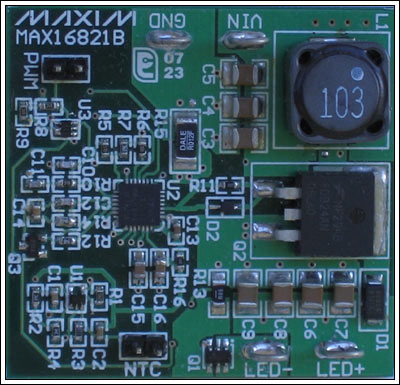More detailed image
(PDF, 1.02MB)
Figure 4. This board contains the LED driver circuit of Figure 1.